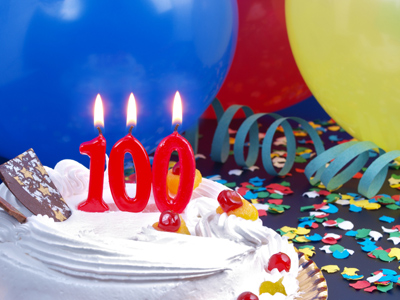If you multiply 0.234 by 100, what number do you get?

# Decimal Numbers (Medium)

Decimal numbers can also be written as fractions. In this 11-plus Maths quiz you are going to get some hands-on practice in writing decimal numbers. Make sure that you LEARN these decimal forms of the fractions because they are used a lot.

Some people get confused when converting decimals to fractions (or fractions to decimals). If you find it difficult, keep on playing our quizzes on decimal numbers until you feel more confident. It really is very simple once you get the hang of it and know the formulae.

Make sure to read the comments after you've answered the questions as these go some way to explaining how the correct answer is arrived at and why.

Enjoy yourself and when you reckon you've cracked it, move onto the next challenge.

1.
If you divide 1.234 by 10, what number do you get?
0.01234
0.1234
0.001234
12.34
If you divide by 10, move the digits one place to the right
2.
If you multiply 0.234 by 10, what number do you get?
0.0234
234.0
23.4
2.34
If you multiply by 10, move the digits one place to the left
3.
What is 'three quarters' as a decimal?
0.85
0.65
0.75
0.25
3/4 = 0.75: divide 3 by 4
4.
If you divide 1.234 by 100, what number do you get?
123.4
0.0001234
0.001234
0.01234
If you divide by 100, move the digits two places to the right
5.
What is 'one half' as a decimal?
0.25
0.5
0.35
0.45
1/2 = 0.5: divide 1 by 2
6.
What is the correct rule for multiplying by a whole number beginning with 1 and followed by one or more zeros, e.g. multiplying by 10, 100, 1,000, ... ?
When multiplying by such a number, move the digits to the left by the same number of zeros as in the number
When multiplying by such a number, move the digits to the right by the same number of zeros as in the number
When multiplying by such a number, move the digits to the left by the same number of digits as in the number
When multiplying by such a number, move the digits to the right by the same number of digits as in the number
For example, 1,000 × 0.234 = 234: the digits have moved three places to the left = the number of zeros in one thousand. The rule for division is as follows: When dividing by such a number, move the digits to the right by the same number of zeros as in the number, e.g. 1.234 ÷ 1,000 = 0.001234: the digits have moved three places to the right = the number of zeros in one thousand
7.
What is 'one tenth' as a decimal?
0.01
0.1
0.001
0.0001
1/10 = 0.1: divide 1 by 10
8.
If you multiply 0.234 by 100, what number do you get?
23.4
2,340
23,400
0.00234
If you multiply by 100, move the digits two places to the left
9.
What is 'one quarter' as a decimal?
0.35
0.45
0.25
0.5
1/4 = 0.25: divide 1 by 4
10.
What is 'one eighth' as a decimal?
0.135
0.145
0.125
0.165
1/8 = 0.125: divide 1 by 8
Author:  Frank Evans